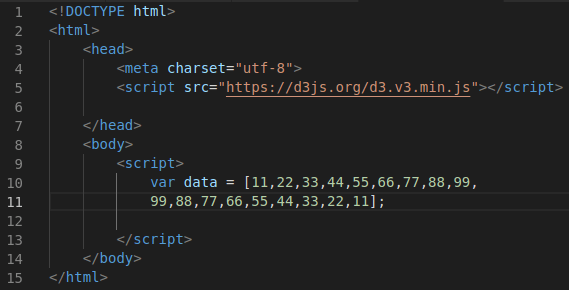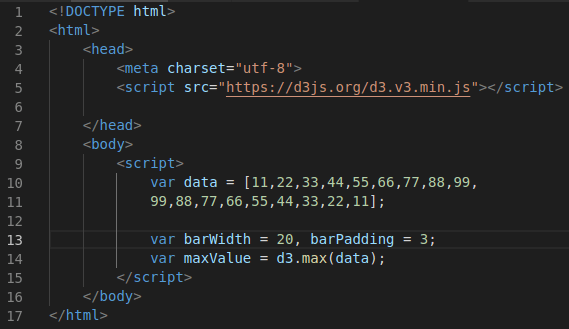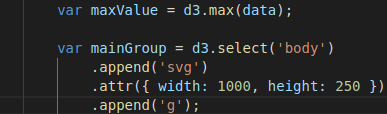# Making Bar Graphs with D3.js

This post will provide an example of how to make a basic bar graph using d3.js.Visualizing data is important and developing bar graphs in one way to communicate information efficiently.

This post has the following steps

1. Initial Template
2. Enter the data
3. Setup for the bar graphs
4. Svg element
5. Positioning
6. Make the bar graph

Initial TemplateBelow is what the initial code should look like.Entering the Data

For the data we will hard code it into the script using an array. This is not the most optimal way of doing this but it is the simplest for a first time experience.  This code is placed inside the second script element. Below is a picture.The new code is in lines 10-11 save as the variable data.

Setup for the Bars in the Graph

We are now going to create three variables. Each is explained below

• The barWidth variable will indicate ho wide the bars should be for the graph
• barPadding variable will put space between the bars in the graph. If this is set to 0 it would make a histogram
• The variable maxValue scales the height of the bars relative to the largest observation in the array. This variable uses the method .max() to find the largest value.

Below is the code for these three variablesThe new information was added in lines 13-14

SVG Element

We can now begin to work with the svg element. We are going to create another variable called mainGroup. This will assign the svg element inside the body element using the .select() method. We will append the svg using .append and will set the width and height using .attr. Lastly, we will append a group element inside the svg so that all of our bars are inside the group that is inside the svg element.

The code is getting longer, so we will only show the new additions in the pictures with a reference to older code. Below is the new code in lines 16-19 directly  under the maxValue variable.Positioning

New=x we need to make three functions.

• The first function will calculate the x location of the bar graph
• The second function  will calculate the y location of the bar graph
• The last function will combine the work of the first two functions to place the bar in the proper x,y coordinate in the svg element.

Below is the code for the three functions. These are added in lines 21-25The xloc function starts in the bottom left of the mainGroup element and adds the barWidth plus the barPadding to make the next bar. The yloc function starts in the top left and subtracts the maxValue from the given data point to calculate the y position. Lastly, the translator combines the output of both the xloc and the yloc functions to position bar using the translate method.

Making the Graph

We can now make our graph. We will use our mainGroup variable with the .selectAll method with the rect argument inside. Next, we use .data(data) to add the data, .enter() to update the element, .append(“rect”) to add the rectangles. Lastly, we use .attr() to set the color, transformation, and height of the bars. Below is the code in lines 27-36 followed by actual bar graph.The graph is complete but you can see that there is a lot of work that needs to be done in order to improve it. However, that will be done in a future post.